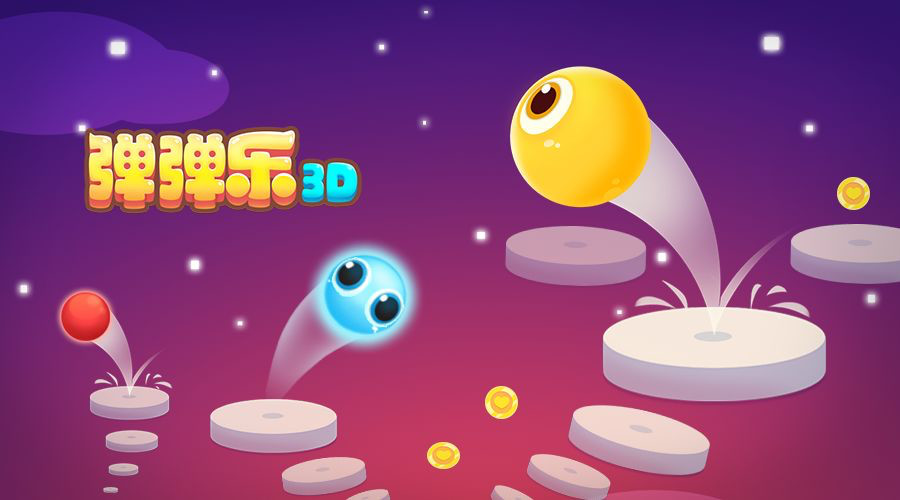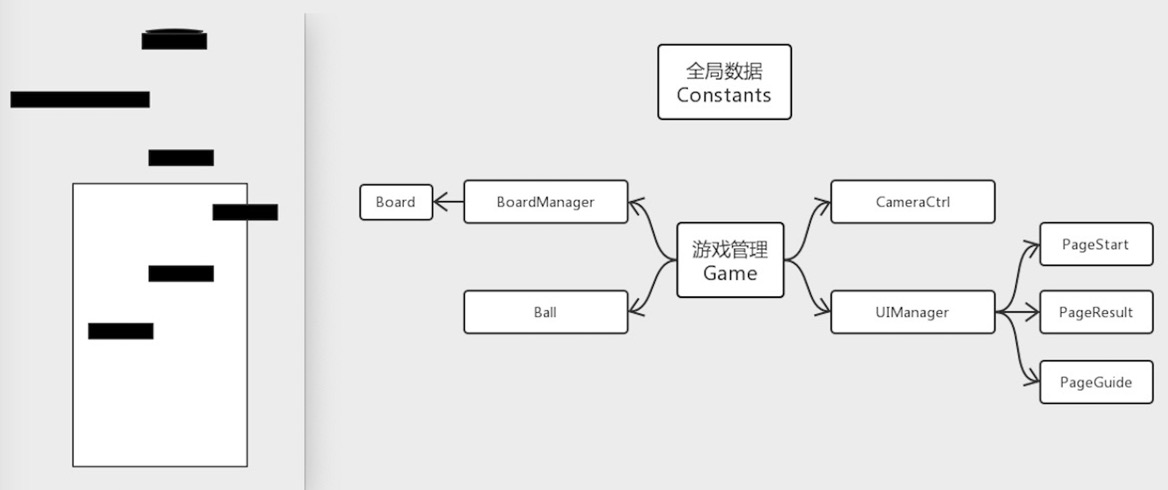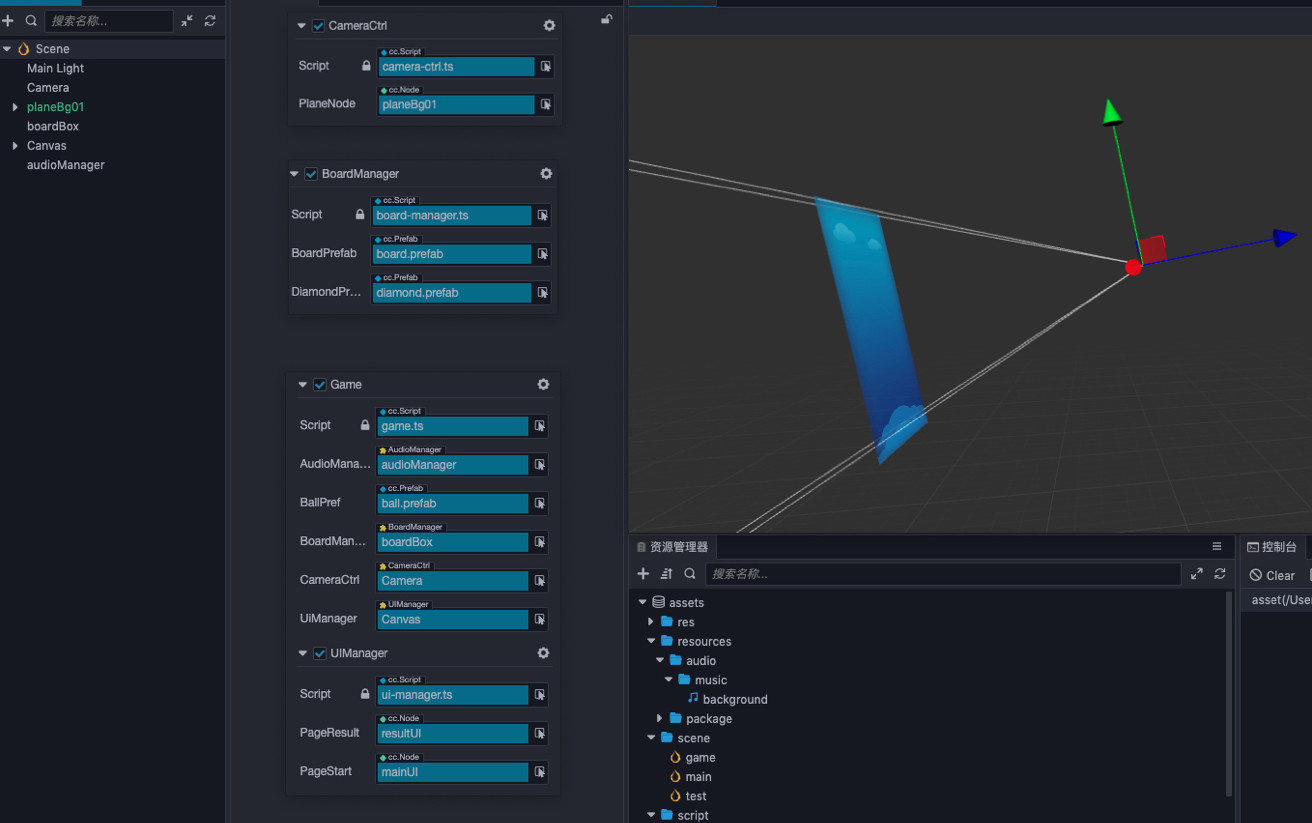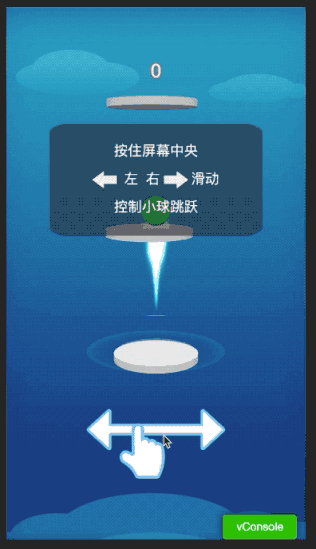# 置顶【Cocos官方 3D 教程】弹弹乐教程和源码分享精选热门1. 游戏介绍

2. 结构说明1. 游戏初始化

- 跳板初始化

- 屏幕事件监听，小球与普通板块弹跳计算

- 提供相机跟随接口

2. 游戏核心逻辑编写

- 跳板复用逻辑编写

- 小球与不同板块弹跳计算

- 游戏开始与结束逻辑编写

3. 游戏丰富

- 添加钻石以及吃砖石表现

- 添加跳板表现

- 增加小球粒子以及拖尾表现

- 增加音效和音乐3. 编写游戏内容逻辑

1. 跳板初始化

getNextPos(board: Board, count: number, out ?: Vec3) {

const pos: Vec3 = out ? outset(board.node.position) : board.node.position.clone();

const o = utils.getDiffCoeff(count, , );

pos.x = (Math.random() - .) * Constants.SCENE_MAX_OFFSET_X * o;

if (board.type === Constants.BOARD_TYPE.SPRINT) {

pos.y += Constants.BOARD_GAP_SPRINT;

pos.x = board.node.position.x;

}

if (board.type === Constants.BOARD_TYPE.SPRING) {

pos.y += Constants.BOARD_GAP_SPRING;

} else {

pos.y += Constants.BOARD_GAP;

}

return pos;

getDiffCoeff(e: number, t: number, a: number) {

return (a * e + ) / ( * e + ((a + ) / t - ));

2. 屏幕事件监听，小球与普通板块弹跳计算

start () {

Constants.game.node.on(Node.EventType.TOUCH_START, this.onTouchStart, this);

Constants.game.node.on(Node.EventType.TOUCH_END, this.onTouchEnd, this);

Constants.game.node.on(Node.EventType.TOUCH_MOVE, this.onTouchMove, this);

this.updateBall();

this.reset();

onTouchStart(touch: Touch, event: EventTouch){

this.isTouch = true

this.touchPosX = touch.getLocation().x;

this.movePosX = this.touchPosX;

onTouchMove(touch: Touch, event: EventTouch){

this.movePosX = touch.getLocation().x;

onTouchEnd(touch: Touch, event: EventTouch){

this.isTouch = false

// Constantsstatic BALL_JUMP_STEP = [0.8, 0.6, 0.5, 0.4, 0.3, 0.2, 0.15, 0.1, 0.05, 0.03]; // 正常跳跃步长static BALL_JUMP_FRAMES = 20; // 正常跳跃帧数

//Ball_tempPos.setthis.node.position);

_tempPos.y += Constants.BALL_JUMP_STEP[Math.floorthis._currJumpFrame / )];

this.node.setPosition(_tempPos);

3. 提供相机跟随接口

update() {

_tempPos.setthis.node.position);

if (_tempPos.x === this._originPos.x && _tempPos.y === this._originPos.y) {

return

}

// 横向位置误差纠正

if (Math.abs_tempPos.x - this._originPos.x) <= Constants.CAMERA_MOVE_MINI_ERR) {

_tempPos.x = this._originPos.x;

this.setPosition(_tempPos);

} else {

const x = this._originPos.x - _tempPos.x;

_tempPos.x += x / Constants.CAMERA_MOVE_X_FRAMES;

this.setPosition(_tempPos);

}

_tempPos.setthis.node.position);

// 纵向位置误差纠正

if (Math.abs_tempPos.y - this._originPos.y) <= Constants.CAMERA_MOVE_MINI_ERR) {

_tempPos.y = this._originPos.y;

this.setPosition(_tempPos);

} else {

const y = this._originPos.y - _tempPos.y;

if (this.preType === Constants.BOARD_TYPE.SPRING) {

_tempPos.y += y / Constants.CAMERA_MOVE_Y_FRAMES_SPRING;

this.setPosition(_tempPos);

} else {

_tempPos.y += y / Constants.CAMERA_MOVE_Y_FRAMES;

this.setPosition(_tempPos);

}

}

1. 跳板复用逻辑编写

for (let i = this.currBoardIdx + ; i >= ; i--) {

const board = boardList[i];

// 超过当前跳板应该弹跳高度，开始下降

if (this.jumpState === Constants.BALL_JUMP_STATE.FALLDOWN) {

if (this.currJumpFrame > Constants.PLAYER_MAX_DOWN_FRAMES || this.currBoard.node.position.y - this.node.position.y > Constants.BOARD_GAP + Constants.BOARD_HEIGTH) {

Constants.game.gameDie();

return

}

// 是否在当前检测的板上

if (this.isOnBoard(board)) {

this.currBoard = board;

this.currBoardIdx = i;

this.activeCurrBoard();

break

}

}

// 当超过中间板就开始做板复用for (let l = this.currBoardIdx - Constants.BOARD_NEW_INDEX; l > ; l--) {

this.newBoard();

2. 小球与不同板块弹跳计算

3. 游戏开始与结束逻辑编写

1. 添加钻石以及吃砖石表现

if (this.type === Constants.BOARD_TYPE.GIANT) {

for (let i = ; i < ; i++) {

this.diamondList[i].active = true

this.hasDiamond = true

}

} elseif (this.type === Constants.BOARD_TYPE.NORMAL || this.type === Constants.BOARD_TYPE.DROP) {

if (Math.random() > .) {

this.diamondList[].active = true

this.hasDiamond = true

}

getNode(prefab: Prefab, parent: Node) {

let name = prefab.data.name;

this.dictPrefab[name] = prefab;

let node: Node = null

if (this.dictPool.hasOwnProperty(name)) {

//已有对应的对象池

let pool = this.dictPool[name];

if (pool.size() > ) {

node = pool.get();

} else {

node = instantiate(prefab);

}

} else {

//没有对应对象池，创建他！

let pool = new NodePool();

this.dictPool[name] = pool;

node = instantiate(prefab);

}

node.parent = parent;

return node;

putNode(node: Node) {

let name = node.name;

let pool = null

if (this.dictPool.hasOwnProperty(name)) {

//已有对应的对象池

pool = this.dictPool[name];

} else {

//没有对应对象池，创建他！

pool = new cc.NodePool();

this.dictPool[name] = pool;

}

pool.put(node);

2. 添加跳板表现和增加小球粒子以及拖尾表现

3. 增加音效和音乐

playSound(play = true) {

if (!play) {

this.audioComp.stop();

return

}

this.audioComp.clip = this.bg;

this.audioComp.play();

playClip() {

this.audioComp.playOneShot(this.click);【本文使用图片素材转载自Cocos官方】